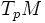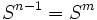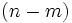# Gauss-Weingarten map

Jump to: navigation, search

## Definition

Suppose$M$ is a differential manifold of dimension$m$, embedded smoothly inside$\R^n$.

### For non-oriented submanifolds

If$M$ is not assumed to have an orientation, the Gauss-Weingarten map is a map from$M$ to the Grassmannian manifold of$m$-dimensional subspaces of$\R^n$, as follows: any point$p \in M$ is mapped to the vector subspace of$\R^n$ parallel to the tangent space$T_pM$.

### For oriented submanifolds

If we give an orientation to$M$ the Gauss-Weingarten map is a map from$M$ to the oriented Grassmannian manifold of$m$-dimensional subspaces of$\R^n$ as follows: any point$p \in M$ is sent to the vector subspace parallel to the tangent space$T_p M$, equipped with the orientation.

### For codimension one submanifolds

For codimension one oriented submanifolds, we can identify the oriented Grassmannian with the sphere$S^{n-1} = S^m$, whereas for codimension one non-oriented submanifolds, we can identify the Grassmannian with real projective space$\R\mathbb{P}^m$.

### Tangent space can be replaced by normal space

We can define the Gauss-Weingarten map, instead, by sending each point to its normal space (the orthogonal complement of the tangent space to$M$ in the tangent space to$\R^n$) with suitable orientation, if the manifold is oriented. The two maps are equivalent, under the isomorphism between the Grassmannian of$m$-dimensional subspaces, and the Grassmannian of$(n-m)$-dimensional subspaces.